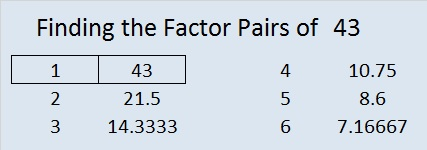# 43 and Find the Factors

• 43 is a prime number.
• Prime factorization: 43 is prime.
• The exponent of prime number 43 is 1. Adding 1 to that exponent we get (1 + 1) = 2. Therefore 43 has exactly 2 factors.
• Factors of 43: 1, 43
• Factor pairs: 43 = 1 x 43
• 43 has no square factors that allow its square root to be simplified. √43 ≈ 6.5574How do we know that 43 is a prime number? If 43 were not a prime number, then it would be divisible by at least one prime number less than or equal to √43 ≈ 6.6. Since 43 cannot be divided evenly by 2, 3, or 5, we know that 43 is a prime number.

43 is never a clue in the FIND THE FACTORS puzzles.

So far today FIND THE FACTORS has received nearly four times as many views as its previous high. When I investigated why I discovered one of the reasons was a blog called mathematicsgames.wordpress.com. On that blog was this post recommending the FIND THE FACTORS puzzles. I am excited for the great write-up and the new people who have looked at the puzzles and used the excel file I made! I am humbled that mathematicsgames wrote the post, and I’m also quite proud to tell you about it.